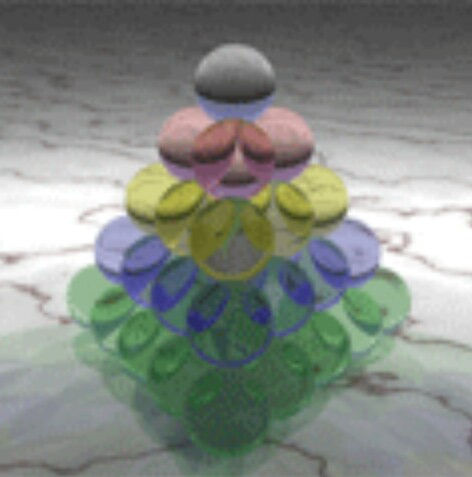top of page
Search

# Tetrahedral numbers sacred geometryTetrahedral tetractys:

1

4

10

20

1+4+10+20=35=(3:5=15:17)=6:8

Tetractys=1+3+6+10=20=(2:0=2:12)

2:12=212:

Enneagram=(star tetrahedron)=(tree of life(E8xE8))=tetragrammaton=tetractys=120-cell

120-cell=120 and 120 is a tetrahedral number

Tetractys=212

6:8=68:

(Enneagram(3D))=(star tetrahedron(3D))=(tree of life(3D))=(tetragrammaton(3D))=(tetrahedral tetractys)=(120-cell ( 5 dimension))

(Tetrahedral tetractys)=68

2³=8

Cosmic tetractys:(Fruit of life Star tetrahedral tetractys(3D cosmic tetractys)):

The cosmic tetractys is 28 circles

so the 3D cosmic tetractys is 84 spheres

so the star tetrahedral tetractys is 155 spheres

So the fruit of life star tetrahedral tetractys is 310 spheres

2x84=168=1:68=68